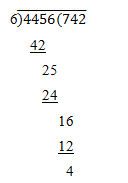# Number Systems ( 6 to 10 )

#### Prev     Next

Number Systems ( 6 to 10 ):

# Aptitude Questions (Number Systems):

6. 6589 – ? = 3434

A)    3242

B)    3155

C)    1123

D)    11023

E)    None of these

Explanation:

Let 6589 – x = 3434

Then, x = 6589 – 3434 = 3155

7. 3251 + 587 + 369 – ? = 3007

A)    1350

B)    1300

C)    1275

D)    1200

E)    None of these

Explanation:

• 3251 + 587 + 369 = 4207
• Let 4207 – x = 3007
• Then, x = 4207 – 3007 = 1200

8.Which of the following is a prime number?

A)    33

B)    81

C)    93

D)    97

E)    None of these

Explanation:

97 are not divisible by any of the prime number 2, 3, 5, 7, 11. Therefore, 97 is a prime number.

9. Which one of the following cannot be the square of a natural number?

A)    40976

B)    55625

C)    38561

D)    143642

E)    None of these

Explanation:

The square of a natural number never ends in 2, 3, 7 or 8.

∴ 143642 is not the square of a natural number.

10. What smallest number should be added to 4456 so that the sum is completely divisible by 6?

A)    7

B)    2

C)    3

D)    4

E)    None of these

Explanation:Required Number = 6 – 4 = 2